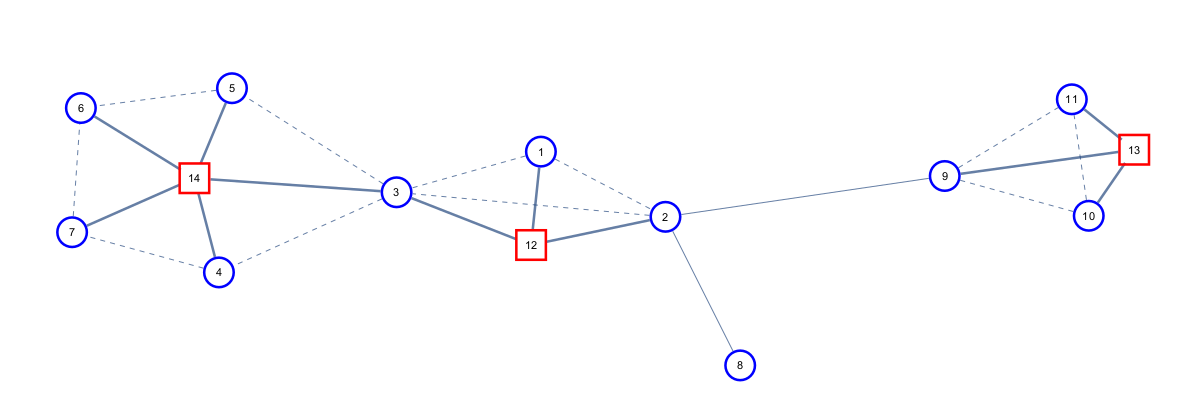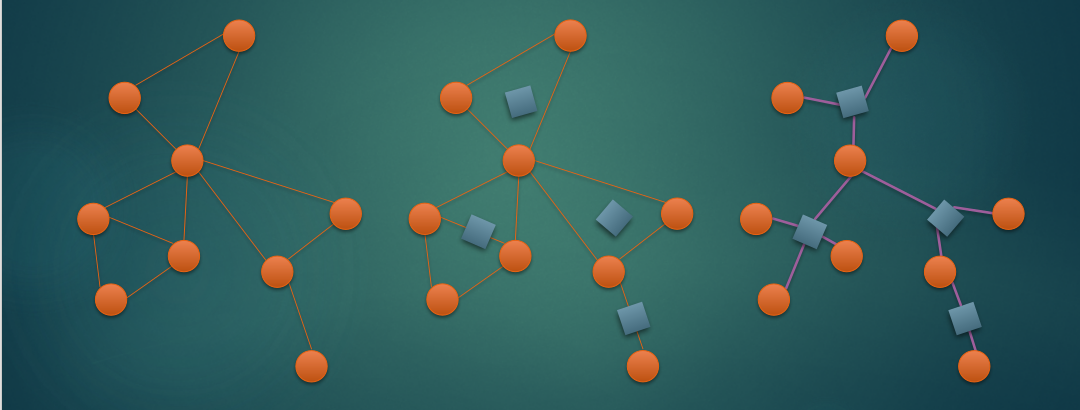# 仙人掌&圆方树学习笔记

## 1、仙人掌

——处理仙人掌的问题。(图片来自于$BZOJ1023$)

——也就是任意一条边只会出现在一个环里面。(图片来源于网络)

## 3、圆方树是啥

$V_ T = R_ T ∪ S_ T , R_ T = V, R_ T ∩ S_ T = ∅$,我们称$R_ T$ 集合为圆点、$S_ T$

$∀e ∈ E$,若 $e$ 不在任何简单环中,则 $e ∈ E_T$

$(p _R , p) ∈ E_ T$ ,即对每个环建方点连所有点

（以下内容来自于课件）

$|V _T | = |S _T | + |R_ T | = |V| + |E| − |V| + 1 = |E| + 1,|E _T | = |E|$

(大小为$r$ 的环在仙人掌和圆方树中都是 $r$ 条边),因此满足 $|V_ T | = |E_ T | + 1$## 5、圆方树的性质

1.方点不会直接和方点相连

2.无论取哪个点为根，圆方树的形态是一样的

$r$为根的仙人掌上的点$p$的子仙人掌是去除掉$p$$r$的所有简单路径后，$p$所在的联通块

3.以$r$为根的仙人掌上$p$的子仙人掌就是圆方树中以$r$为根时，$p$子树中的所有圆点

## 7、广义圆方树### 带修改，求无向图中两点之间所有简单路径上的最小权值(CF487E)

(为什么要手写可删堆啊?$multiset$不好吗？)

## 8、完结撒花

posted @ 2018-05-28 08:09 小蒟蒻yyb 阅读(...) 评论(...) 编辑 收藏